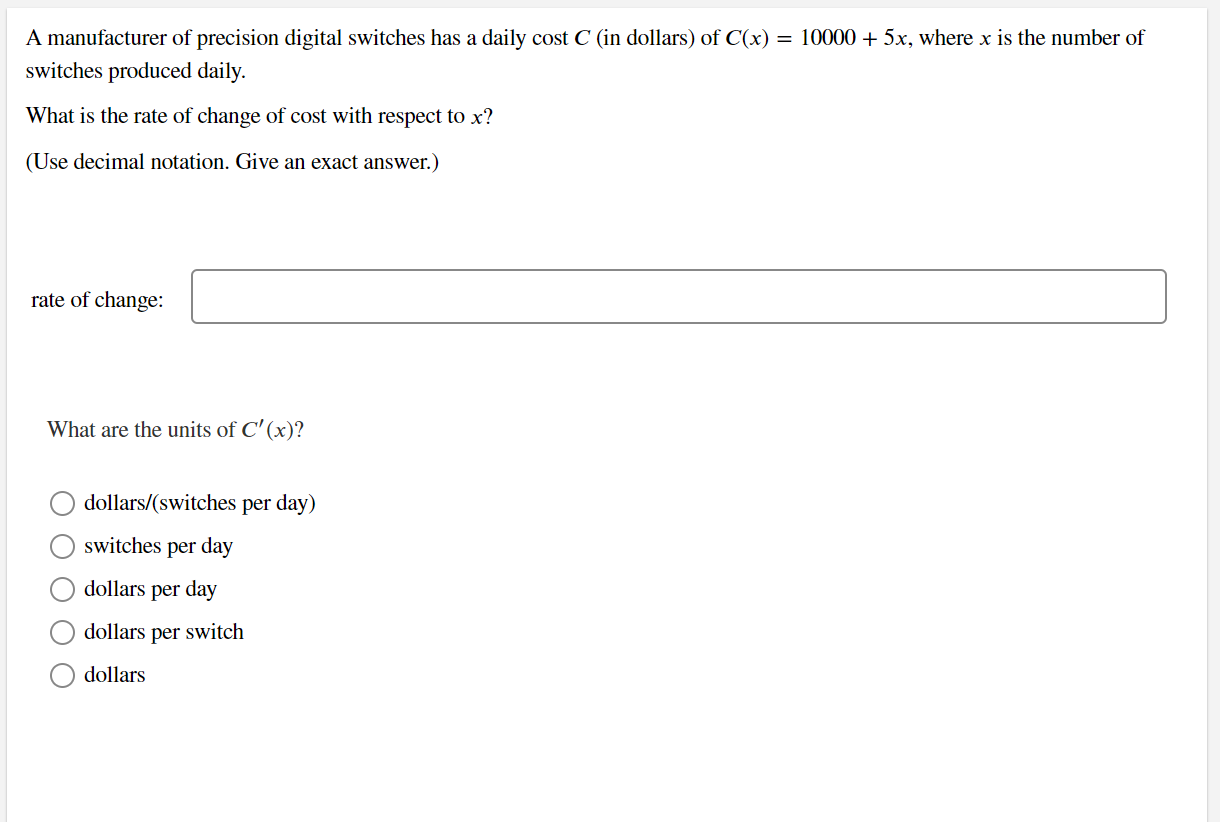# Question Solved1 AnswerA manufacturer of precision digital switches has a daily cost 𝐶 (in dollars) of 𝐶(𝑥)=10000+5𝑥, where 𝑥 is the number of switches produced daily. What is the rate of change of cost with respect to 𝑥? (Use decimal notation. Give an exact answer.) A manufacturer of precision digital switches has a daily cost C (in dollars) of C(x) = 10000 + 5x, where x is the number of switches produced daily. What is the rate of change of cost with respect to x? (Use decimal notation. Give an exact answer.) rate of change: What are the units of C'(x)? dollars/(switches per day) switches per day dollars per day dollars dollars per switchA manufacturer of precision digital switches has a daily cost 𝐶 (in dollars) of 𝐶(𝑥)=10000+5𝑥, where 𝑥 is the number of switches produced daily. What is the rate of change of cost with respect to 𝑥? (Use decimal notation. Give an exact answer.)Transcribed Image Text: A manufacturer of precision digital switches has a daily cost C (in dollars) of C(x) = 10000 + 5x, where x is the number of switches produced daily. What is the rate of change of cost with respect to x? (Use decimal notation. Give an exact answer.) rate of change: What are the units of C'(x)? dollars/(switches per day) switches per day dollars per day dollars dollars per switch
More
Transcribed Image Text: A manufacturer of precision digital switches has a daily cost C (in dollars) of C(x) = 10000 + 5x, where x is the number of switches produced daily. What is the rate of change of cost with respect to x? (Use decimal notation. Give an exact answer.) rate of change: What are the units of C'(x)? dollars/(switches per day) switches per day dollars per day dollars dollars per switch